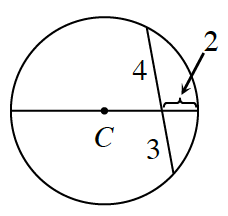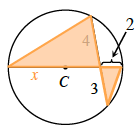### Home > GC > Chapter 11 > Lesson 11.1.3 > Problem11-43

11-43.Find the area and circumference of $⊙C$ at right. Show all work.

The two triangles are similar, which means the ratios of corresponding sides are equal.

Therefore $\frac{2}{4} = \frac{3}{x} \rightarrow x=6$

Since you now know that $x = 6$, you can determine the diameter and radius of the circle.$\text{Radius}=4$

$\text{Area} = 50.27 \; \text{square units}$

$\text{Circumference} = 25.13 \; \text{units}$